# A brief introduction to statistical modeling

Here I talk a lot about “the model” as if it’s a living, breathing thing. But there isn’t anything mysterious about a model, it’s a totally understandable thing based on math, data, and a bunch of assumptions.

When you think about a “model” used in regular life, it’s something like a scale model of a building. Why is such a thing useful? After all, the model of a building isn’t functional in any of the same ways as the building itself. Nobody can live in it, go inside it. The model is useful, though, to a group of architects trying to lay out a neighborhood, or to a plumber deciding where to run his pipes. That is, a model is purposeful: it only has to be like the real system in a way that you get the information you need.

When you build a numerical model, you’re building a description of something. Consider the first thing most physics students learn: the path a ball takes when you toss it into the air.

Suppose we have video footage of a ball being tossed into the air. Using the video, we write down the position of the ball along the horizontal and vertical on each video frame, and we plot them. We might get something that looks like this: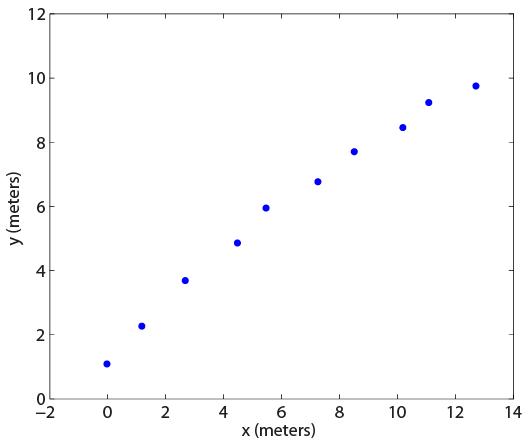To make a model of the data, we want to describe the data. In this case, we might choose something natural, like fitting a curve to the data points. To start, I might choose a linear regression, meaning that a line in the x-y plane is drawn that most closely approximates the points.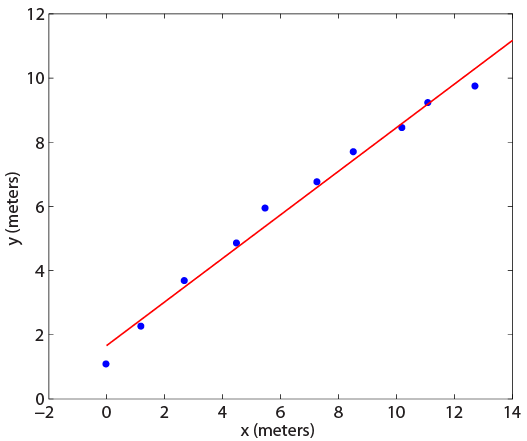This curve fit is actually quite good. It has an R2 value of 0.98, where the best possible value is 1.00. That roughly means this description (the linear one) is 98% complete. There is still some jitter, so the line doesn’t neatly go through each point, but it’s nonetheless quite a good description of the data.

The only problem is that it’s utter nonsense. The trajectory that a ball takes when you throw it is not a line. This comes into relief particularly if we want to use our model to project or extrapolate. To do this, we would see what y-value this line had at x-values beyond x=14. For instance, someone might ask us where the ball fell to the ground. But this linear model would say that the ball would just go on forever, eventually escaping the Earth’s gravity, hurtling into space, and never hitting the ground! Our model has no predictive power because it isn’t predicated on any common sense. When it comes to model selection, you have to choose something reasonable. In this case, we at least need a curve that can bend down, since we know eventually the ball will hit the ground.

The next natural approximation would be a parabola.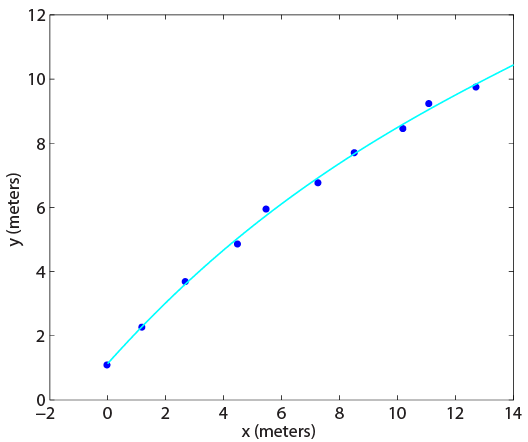The fitting statistic now is 0.998, meaning this is a very good fit to the data, but so was the line. And a cubic curve is even better. However, their predictions are quite different.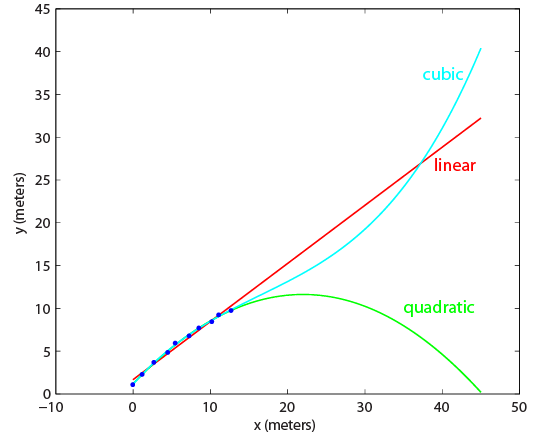The linear model, as I said, predicts that the ball just sails forward and upward to infinity. The quadratic (parabola) says that the ball reaches an apex around 23 meters to the right of where it was thrown, and then lands about 45 meters away from the person who threw it. The cubic model predicts that it turns upwards and sails nearly straight up!

Because this is physics, we know what the right answer is. Assuming a linear gravitational field, the equations of motion can be solved exactly, and the correct answer is parabolic. So, when you set out to model something like this, you can use your prior information to choose a good model. Note, however, that even this model might fail if there is a lot of turbulence, drag forces, or if the projectile is moving very fast (so that the Coriolis force is important). That is, even the “correct” model is subject to some stipulations.

Why do we have to do model selection in this way? If physics says the path is parabolic, then we should be able to fit a curve straight through all of the data points. The reason is that there are fluctuations, or noise, in the data. This noise might be physical (a gust of wind blew the ball a little upwards at one point) or it might be measurement-related (the camera recording the ball shifted a little, or the film moved left to right as the shutter opened). Whatever the reason, the model will always be fit with some error. If there were no error in the above data, then a cubic would have fit exactly as well as a quadratic, and the coefficient of the cubic term would have been 0. In reality, the cubic coefficient was 0.0007, low, but nonzero, and hence it gave a wildly wrong prediction.

The cubic in this example is overfit. It tried to make too much of the noise in the data, and predicted a path which is obviously wrong. The linear fit is underfit: it is oversimplified and ignores reasonable expectations. These are pitfalls that have no technical rules governing them (This is not entirely true if you take a Bayesian point of view rather than a frequentist one. However, that discussion is beyond the scope of this article.). That is, there is no rule that if you follow it you get the right model.

Fitting a curve to a set of known data is called regression analysis, because it finds the least-squares regression of a given data set. Once you have a reasonable fit, if your model is good, you can then plug new numbers in as the input variable (x, in this example) and get a prediction of what value (y value, height) the system would be expected to take on.

There are any number of curves available for regression analysis. In addition to polynomial fits (as the projectile example used), exponential or logarithmic functions are commonly used. The most well known stats curve of all is called a Gaussian or normal curve, and is the exponential with a quadratic in the exponent (this makes the bell curve that matches so many phenomena). Nearly all polling data relies on the properties of this curve, as does the grading in many college classes. (You can read my earlier discussion of how polling works here: part 1 and part 2)

A regression can take several input variables (called explanatory variables) to return one output (response variable). For instance, in the model of a ball thrown into the air, a second variable representing degree of headwind might be incorporated. This allows for a prediction under a wider range of applications.

Let’s take another example, which is somewhat more relevant for this site. Suppose that you want to say whether a certain person is likely to get lung cancer. The first thing you would do is go survey a bunch of people, and maybe you would ask them just three questions: 1. How many cigarettes do you typically smoke in a week? 2. How old are you? 3. Have you been diagnosed with lung cancer? In doing this survey you are measuring two explanatory variables (number of cigarettes and age) and the response variable (cancer or no cancer). The response variable is now not continuous, as in projectile motion, but categorical; it only takes on the values of yes or no. The explanatory variables are still continuous, but they don’t have to be. You could also ask “do you have a close family member who had lung cancer?” and use this answer in your analysis (this is frequently called a dummy variable).

Some sample data that I made up is plotted below. We code getting cancer as “1” and not as “0” when we plot.Of course, there are very young people who get lung cancer, and also nonsmokers or light smokers that also do so. However, the people who got cancer tend to be older and people who smoke. The question is, what curve should we try to fit?

There are several that can work, but generally we want something that takes in any number and returns a number between 0 and 1. Then, we can interpret the value of the curve as a probability. A curve that’s sigmoidal (sort of s shaped) would work nicely. After that, it’s just a matter of fitting the odds. The process for doing this is highly complicated (it requires iteration), but if we don’t worry about the process we can generate a fit to the above data.

For a 50 year old man, the probability curve of the logistic regression looks like this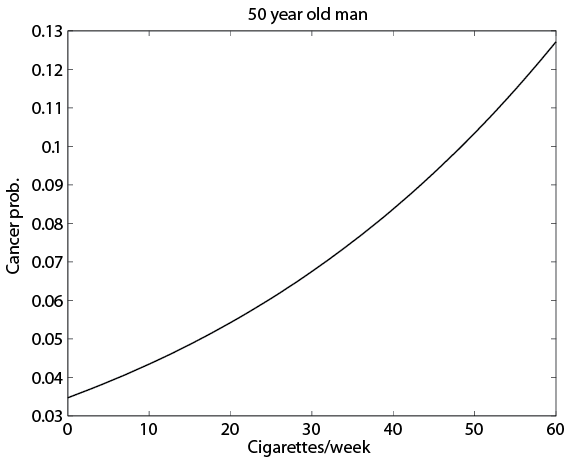According to our (totally fake) data, the probability of a 50 year old nonsmoker getting lung cancer is about 3.5%, but at two packs a day it’s more like 9%, a significant increase. This type of model works quite well for predicting incidences of many things whose outcome is a yes or no proposition.

Regression analysis is only one kind of statistical model, but it’s quite powerful when limited information is available. With a better idea of what is going on, it’s possible to build much more sophisticated models, ones that use simulations with random number generators to determine correlations between outcomes (this is how the model at 538 works). But as a first attempt, most people will try something like the above.

Reuben is the statistician and lead writer at IdolAnalytics. Follow him on Twitter. His personal site (run all year round) is IdleAnalytics
.